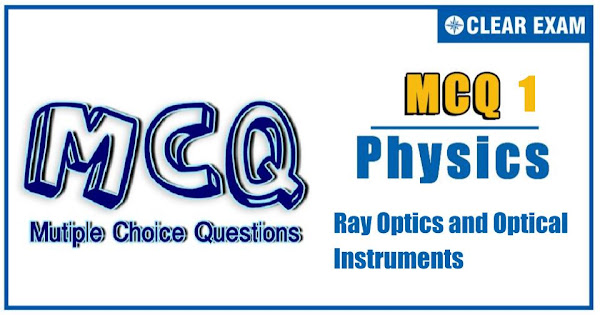## [LATEST]\$type=sticky\$show=home\$rm=0\$va=0\$count=4\$va=0

JEE Advanced Physics Syllabus can be referred by the IIT aspirants to get a detailed list of all topics that are important in cracking the entrance examination. JEE Advanced syllabus for Physics has been designed in such a way that it offers very practical and application-based learning to further make it easier for students to understand every concept or topic by correlating it with day-to-day experiences. In comparison to the other two subjects, the syllabus of JEE Advanced for physics is developed in such a way so as to test the deep understanding and application of concepts.

Q1. A bird is flying up at angle sin^(-1)⁡(3/5) with the horizontal. A fish in a pond looks at that bird. When it is vertically above the fish. The angle at which the bird appears to fly (to the fish) is [n_water=4/3]
•  sin^(-1)⁡(3/5)
•  sin^(-1)⁡(4/5)〗
•  45°
•  sin^(-1)⁡(9/16)
Q2.A ray of light passes from glass, having a refractive index of 1.6, to air. The angle of incidence for which the angle of refraction is twice the angle of incidence is:
•  sin^(-1)⁡(4/5)
•  sin^(-1)⁡(3/5)
•  sin^(-1)⁡(5/8)
•  sin^(-1)⁡(2/5)
Q3.  Two convex lenses placed in contact form the image of a distant object at P. If the lens B is moved to the right, the image will
•   Move to the left
•  Move to the right
•  Remain at P
•  Move either to the left or right, depending upon focal lengths of the lenses
Solution
Power of the system decreases due to separation between the lenses. So, the focal length increases.

Q4. A plane glass mirror of thickness 3 cm of material of μ=3/2 is silvered on the back surface. When a point object is placed 9 cm from the front surface of the mirror, then the position of the brightest image from the front surface is
•  9 cm
•  11 cm
•  12 cm
•  13 cm
Solution
A thick glass mirror produces a number of images There is an apparent shift of actual silvered surface toward the unsilvered face Effective distance of the reflecting surface from unsilvered face =d/μ=3/(3/2) cm=2 cm Distance of the point object from effective reflecting surface =9 cm+2 cm= 11 cm Distance of image from the point object =11 cm+11 cm=22 cm Distance of image from unsilvered face =(22-9) cm =13 cm

Q5. The graph between object distance uand image distance v for lens is given below. The focal length of the lens is

•  5 ± 0.1
•  5 ± 0.05
•  0.5 ± 0.1
•  0.5 ± 0.05
Q6. A plano-convex lens fits exactly into a plano-concave lens. Their plane surfaces are parallel to each other. If the lenses are made of different material of refractive indices μ_1 and μ_2 and R is the radius of curvature of the curved surface of the lenses, then focal length of the combination is
•  R/(μ1-μ2 )
•  2R/(μ1-μ2 )
• R/2(μ1-μ2 )
•  R/2- (μ1+μ2 )
Q7.Light from a denser medium 1 passes to a rarer medium 2. When the angle of incidence is θ the partially reflected and refracted rays are mutually perpendicular. The critical angle will be
•  sin^(-1)⁡(cot⁡θ)
•  sin^(-1)⁡(tan⁡θ)
•  sin^(-1)⁡(cos⁡θ)
•  sin^(-1)⁡(sec⁡θ)
Q8.Every decision of the Union Cabinet is taken in the nameRefractive index of a prism is √(7/3) and the angle of prism is 60°. The minimum angle of incidence of a ray that will be transmitted through the prism is
•  30°
•  T45°
•  15°
•  50°
Q9.A convex lens of power +6 dioptre is placed in contact with a concave lens of power -4 dioptre. What will be the nature and focal length of this combination?
•  Concave, 25 cm
•  Convex, 50 cm
•  Concave, 20 cm
•  Convex, 100 cm
Q10. Figure (a) shows two plano-convex lenses in contact as shown. The combination has focal length 24 cm. Figure (b) shows the same with a liquid introduced between them. If refractive index of glass of the lenses is 1.50 and that of the liquid is 1.60, the focal length of system in figure(b) will be
•  -120 cm
•  120 cm
•  -24cm
• 24cm
Solution
Since the refractive index of the liquid is greater than the refractive index of glass, therefore the focal length of the system has to be negative. So, options (b) and (d) are excluded Now, 1/24=(1.5-1) 2/R Or R=24 cm Again, for liquid concave lens,   1/f=-(1.6-1)(2/24) 1/f=-3/5×1/12 Or 1/f=-1/20 or f=-20 cm Now, 1/F=1/24-1/20=(5-6)/120 Or F=-120 cm#### Written by: AUTHORNAME

AUTHORDESCRIPTION## Want to know more

Please fill in the details below:

## Latest NEET Articles\$type=three\$c=3\$author=hide\$comment=hide\$rm=hide\$date=hide\$snippet=hide

Name

ltr
item
BEST NEET COACHING CENTER | BEST IIT JEE COACHING INSTITUTE | BEST NEET, IIT JEE COACHING INSTITUTE: Ray Optics and Optical Instruments-Quiz-1
Ray Optics and Optical Instruments-Quiz-1
https://1.bp.blogspot.com/-MSjS17eNPeo/YNw393UfFSI/AAAAAAAALpE/QFAQV9JhxxU99ac-L5BI-bEYIVHbudx6wCLcBGAsYHQ/s600/Quiz%2BImage%2B20.jpg
https://1.bp.blogspot.com/-MSjS17eNPeo/YNw393UfFSI/AAAAAAAALpE/QFAQV9JhxxU99ac-L5BI-bEYIVHbudx6wCLcBGAsYHQ/s72-c/Quiz%2BImage%2B20.jpg
BEST NEET COACHING CENTER | BEST IIT JEE COACHING INSTITUTE | BEST NEET, IIT JEE COACHING INSTITUTE
https://www.cleariitmedical.com/2021/07/ray-optics-and-optical-instruments-quiz.html
https://www.cleariitmedical.com/
https://www.cleariitmedical.com/
https://www.cleariitmedical.com/2021/07/ray-optics-and-optical-instruments-quiz.html
true
7783647550433378923
UTF-8